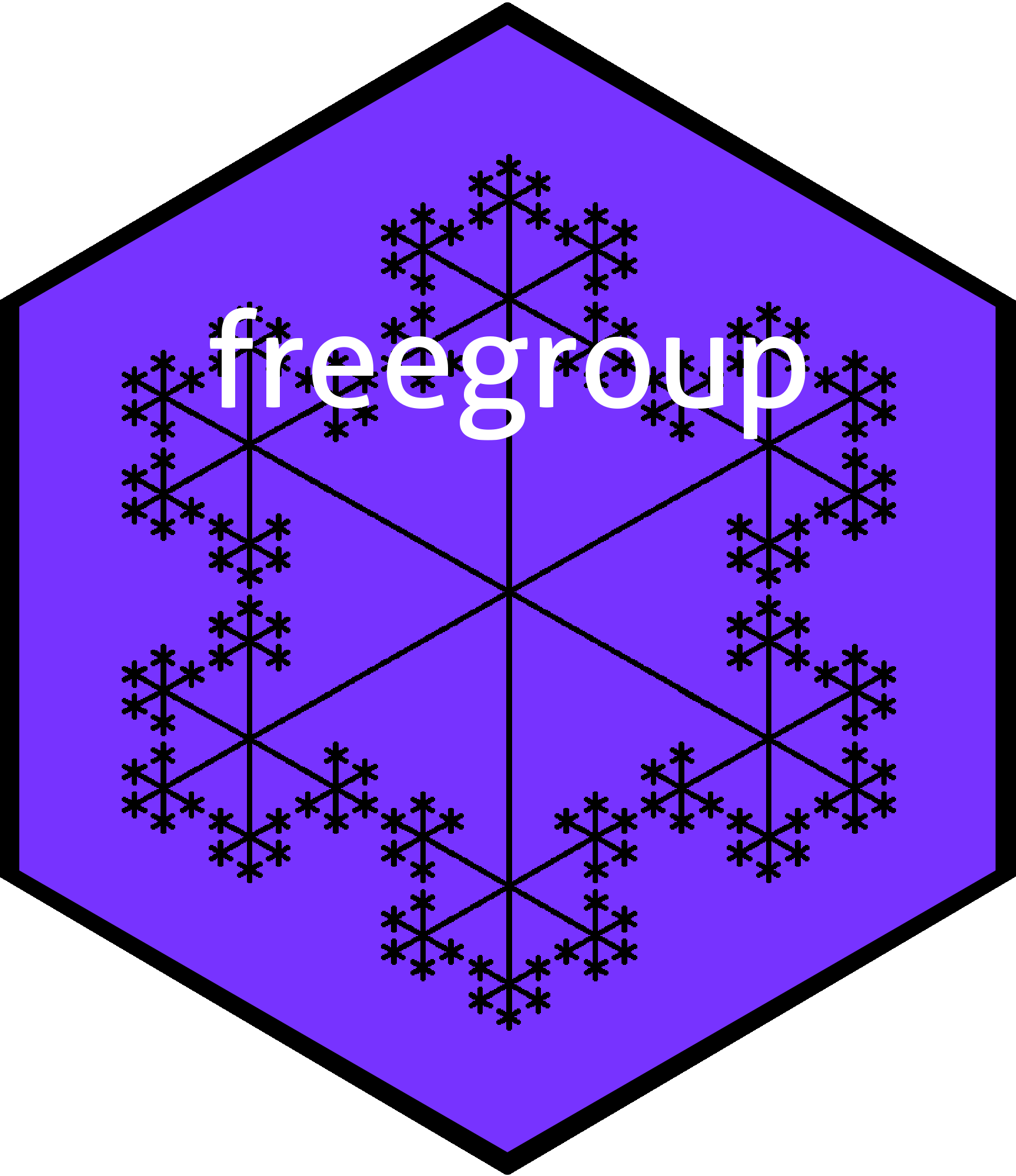# The free group in R# Overview

The `freegroup` package provides functionality for working with the free group in R. A detailed vignette is provided in the package. Informally, the free group is the set of words that are objects like , with a group operation of string juxtaposition. Usually one works only with words that are in ``reduced form’’, which has successive powers of the same symbol combined, so would be equal to ; see how appears to the third power and the term in the middle has vanished.

The group operation of juxtaposition is formally indicated by , but this is often omitted in algebraic notation; thus, for example .

# Installation

You can install the released version of freegroup from CRAN with:

``````# install.packages("freegroup")  # uncomment this to install the package
library("freegroup")``````

# The `freegroup` package in use

Function `rfree()` generates a vector of random free group elements, giving quick “get you going” examples:

``````a <- rfree(10,5)
a
#>   a.d^5.a^-4.b^5.e^-3 a.e^2.b^2           a^3.e^-7.b^-3
#>   b^7.d^4             a^2.b^-4.c^-4       a^-5.d^3.c^3
#>   d^-5.e^-1.d^-5.e^3  c.b^-7.c^4.b^-5     c^6.e^2
#>  e^-3.d^5.a^-5
b <- as.free('x')``````

Then we can perform various operations on these vectors:

``````a+b
#>   a.d^5.a^-4.b^5.e^-3.x a.e^2.b^2.x           a^3.e^-7.b^-3.x
#>   b^7.d^4.x             a^2.b^-4.c^-4.x       a^-5.d^3.c^3.x
#>   d^-5.e^-1.d^-5.e^3.x  c.b^-7.c^4.b^-5.x     c^6.e^2.x
#>  e^-3.d^5.a^-5.x
a-b
#>   a.d^5.a^-4.b^5.e^-3.x^-1 a.e^2.b^2.x^-1           a^3.e^-7.b^-3.x^-1
#>   b^7.d^4.x^-1             a^2.b^-4.c^-4.x^-1       a^-5.d^3.c^3.x^-1
#>   d^-5.e^-1.d^-5.e^3.x^-1  c.b^-7.c^4.b^-5.x^-1     c^6.e^2.x^-1
#>  e^-3.d^5.a^-5.x^-1
a^b
#>   x^-1.a.d^5.a^-4.b^5.e^-3.x x^-1.a.e^2.b^2.x
#>   x^-1.a^3.e^-7.b^-3.x       x^-1.b^7.d^4.x
#>   x^-1.a^2.b^-4.c^-4.x       x^-1.a^-5.d^3.c^3.x
#>   x^-1.d^-5.e^-1.d^-5.e^3.x  x^-1.c.b^-7.c^4.b^-5.x
#>   x^-1.c^6.e^2.x             x^-1.e^-3.d^5.a^-5.x``````

There are a number of package functions that work in a vectorized way:

``````sum(a)
#>  a.d^5.a^-4.b^5.e^-3.a.e^2.b^2.a^3.e^-7.b^4.d^4.a^2.b^-4.c^-4.a^-5.d^3.c^3.d^-5.e^-1.d^-5.e^3.c.b^-7.c^4.b^-5.c^6.e^-1.d^5.a^-5``````

The package also supports extraction and replacement:

``````a[3:9] <- as.free('xy')
a
#>   a.d^5.a^-4.b^5.e^-3 a.e^2.b^2           x.y
#>   x.y                 x.y                 x.y
#>   x.y                 x.y                 x.y
#>  e^-3.d^5.a^-5``````

Various simple elements can be created:

``````alpha(1:10)
#>   a b c d e f g h i j
abc(1:5)
#>  a         a.b       a.b.c     a.b.c.d   a.b.c.d.e``````

# Further information

For more detail, see the package vignette

`vignette("freegroup")`